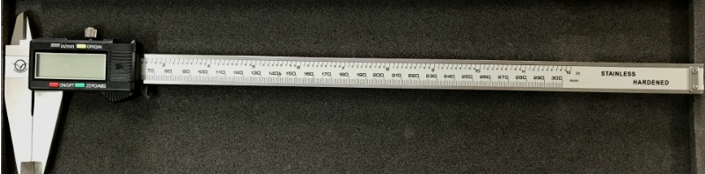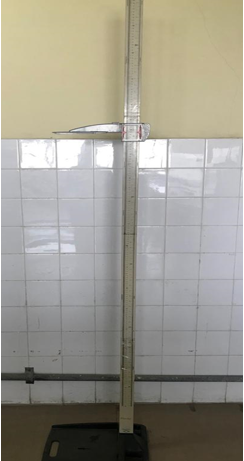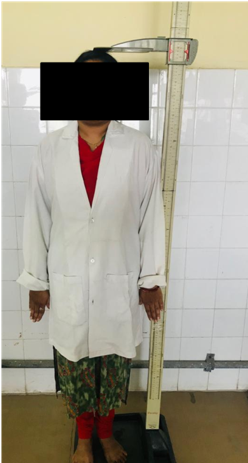Print ISSN:-2394-2118

Online ISSN:-2394-2126

CODEN : IJCAGX

Current Issue

Year 2020

Volume: 7 , Issue: 2

#### Article Access statistics

Viewed: 191

Emailed: 0Parmar, Ruparelia, and Patel: Estimation of height from the lengths of second and fourth digits of hand in Gujarat region

## Introduction

Estimation of stature for personal identification forms a very important criteria in cases of incomplete skeletal and decomposed human parts. 1 Personal identification means determination of individuality of a person. The primary characteristics of identity are age, sex and height. 2 Stature is considered as natural height of a person in upright position. It is maximum between the age group of 20-25 years. 3 It is found that there is a close relationship between the height of the person and various parts of body like head, trunk and lengths of upper and lower limbs; 4,5 estimate of which is considered to be important in those cases where only fragmentary or mutilated remains of unknown persons are recovered.6 Similar type of study has been done on cadavers in past. But limitation is population cannot be represented by cadavers. Most of the times cadavers are of those persons who are aged and suffered from chronic debilitating diseases. Again it is found that cadavers are of persons who have been dying in an abnormal posture and therefore it is very difficult to straighten the body to get accurate stature measurement. 7 In recumbent posture height is increased by 2.5cm after death. Because of constrains mentioned above of cadaveric study, we selected living persons to conduct this study. Although there is strong relationship between body parameters that have been determined, it has been found that the r e is high variation in parametres from population to population and ethnic origin because of differences in nutrition and levels of physical activity.8 Therefore the present study is conducted in the living adults.

We can use alternate parameter (digit length) to measure height which is not possible to measure in cases of age related loss in stature, deformity like kyphosis, scoliosis, lordosis contracture and missing legs.9 The study aims to carry out the relationship between height and 2nd and 4th digits length in living subjects of Gujarat region, to derive a population specific formula (regression equation) to estimate height from 2nd and 4th digit length. We can come to know the probable height of an individual by 2nd and 4th digit length using regression analysis.

## Materials and Methods

Institutional review board has approved my study. A cross sectional sample of 500 (230 males and 270 females) asymptomatic healthy students between age group of 18-24 years belonging to Gujarat region were selected.

### Methods of collection of data

All the study subjects were given adequate information about the study and informed consent is taken. The study method was approved by the institutional review board. We have measured all the parameters in ventilated room during the time between 2:00pm to 5:00pm to avoid diurnal variation. It was measured and recorded only by us. Height, right and left 2nd and 4th digit length of each individual were recorded.

Person having significant disease or deformity that could have affected the general or bony growth are excluded.

Landmarks and techniques involved in taking anthropometric measurements:

#### Height

Standard anthropometer was used to measure height. The person was asked to stand erect on horizontal plane with eyes looking forward, palms of hands turned inwards and fingers pointing downwards and vertical distance from vertex to floor is measured.

#### Digit length

Sliding caliper was used to measure the digit length. The subjects were asked to place their hands supine on a flat hard horizontal surface with fingers extended. It is a linear distance between the proximal crease of digits and the distal end of the most anterior projecting point, i.e., tip of the finger.

### Measurements

Stature (Height) and digit length are measured in centimetres.

Statistical Analysis done by:

1. Simple Linear regression equation........Y=a + bX

Where,

Y= Height of Individual

a = Intercept

b = Regression Coefficient

X = Digit Length

### Outcome Measures

1. Relationship between person’s height and digit length in living subject.

2. Derive a population specific formula to estimate height from digit length.

Statistical Methods used for this study include Mean, Standard deviation, and Standard error of mean, Correlation of coefficient, Regression coefficient, Value of constant and ‘t’ test.

### Sliding Caliper### Standard height measuring instrument### Measurement of stature#### Correlation coefficient (r)

The extent or degree of relationship between two sets of figures is measured in terms of other parameters called Person’s Correlation coefficient. It is denoted by letter ‘ r ’.

r=n(xy)-(x)(xy)nx2-(x)2ny2-(y)2

#### Regression coefficient (b):

Regression coefficient of Y on X is denoted as byx and that of X on Y as bxy.

byx=xy-xynx2-x2n

bxy=xy-xyny2-y2n

#### Value of constant (a)

It is denoted by ‘ a ’. It is intercept of Regression line. It is derived from as:

a=Y--bX-

Simple linear Regression formula: Y = a+bX

Y = Height

X = Length of Right or Left hand digit

a = Intercept/ constant

b = Regression coefficient

Y1 = a + bXr (Right)

Y2 = a + bXl (Left)

## Result

The observations and statistical outcomes of the study are presented in the tables.

##### Table 1
 Sr. No Variable Height (cm) Right 2nd Digit (cm) Right 4th Digit (cm) Left 2nd Digit (cm) Left 4th Digit (cm) 1 Minimum 155 5.9 6.2 4.4 6.2 2 Maximum 185 8.4 8.6 8.4 8.6 3 Mean/ Average 170.04 7.30 7.48 7.39 7.44 4 Standard Deviation (SD) 6.07 0.40 0.42 0.47 0.43 5 Coefficient of Variation (CV) 3.56 5.52 5.70 6.42 5.81

#### Descriptive statistics of parameters studied in Male

##### Table 2
 S. No Variable Height (cm) Right 2nd Digit (cm) Right 4th Digit (cm) Left 2nd Digit (cm) Left 4th Digit (cm) 1 Minimum 136 5.9 5.9 5.9 5.9 2 Maximum 176 7.8 8.1 7.8 7.9 3 Mean/ Average 157.12 6.69 6.82 6.75 6.78 4 Standard Deviation (SD) 5.72 0.34 0.38 0.36 0.40 5 Coefficient of Variation ( CV) 3.64 5.14 5.63 5.42 5.91

#### Descriptive statistics of parameters studied in female

##### Table 3
 S. No. Independent Variable Right Hand 2nd Digit Right Hand 4th Digit Left Hand 2nd Digit Left Hand 4th Digit 1 Correlation Coefficient (r) 0.100 0.548 0.14 0.53 2 Coefficient of Determination (r2 ) 0.01 0.29 0.019 0.28 3 Regression Coefficient (b) 1.51 7.79 1.90 7.50 4 Intercept (a) 158.95 111.67 155.98 114.13

#### Person’s correlation coefficient in males

##### Table 4
 S.No. Independent Variable Right Hand 2nd Digit Right Hand 4th Digit Left Hand 2nd Digit Left Hand 4th Digit 1 Correlation Coefficient (r) 0.319 0.594 0.308 0.466 2 Coefficient of Determination (r2 ) 0.101 0.352 0.094 0.217 3 Regression Coefficient (b) 5.31 8.82 4.81 6.65 4 Intercept (a) 121.57 96.83 124.59 111.9

#### Person’s correlation coefficient in females

##### Table 5
 Simple Linear Regression Formula Males and Females (500) For Right Hand For Left Hand Y = a + bX Yr-2 = 91.12 + 10.34Xr-2 Yl-2 = 160.28 + 0.44Xl-2 Y = a + bX Yr-4 = 72.05 + 12.78X r-4 Yl-4 = 78.72 + 11.91Xl-4

#### Regression analyses for prediction of height in all cases

[i] Where, Y = Height

[ii] Xr-2 = Right hand 2nd digit length

[iii] Xr-4 = Right hand 4th digit length

[iv] Xl-2 = Left hand 2nd digit length

[v] Xl-4 = Right hand 4th digit length

Value of Correlation coefficient (r) in male: Height and right 2nd digit & height and 4th digit length is 0.100 and 0.548 respectively. Height and left 2nd digit & height and 4th digit length is 0.140 and 0.5 30 respectively.

Value of Correlation coefficient (r) in female: Height and right 2nd digit & height and 4th digit length is 0.319 and 0.5 94 respectively. Height and left 2nd digit & height and 4th digit length is 0.308 and 0.466 respectively.

## Discussion

In addition to the various linear measurements efforts have been made by various workers to find out Correlation between height and digits’ length in different regions. The present study deals with the observation on correlation of height (stature) with 2nd and 4th digits length in Gujarat region.

The height of an individual mainly being genetically predetermined is an inherent characteristic, the estimate of which is considered to be an important in the identification of unknown human remains.10

Height estimation formulae based on digit length shows similar level of accuracy for calculation based on the length of other long bones.11 We selected these criteria because compared to other bones of the body; it is easier to get more accurate measure of digit length in living subjects. The values have become increasingly important in the identification of persons.

The result of the present study shows that the dimensions of the digits can successfully be used for estimations of stature by law enforcement agencies and forensic scientists. The only precautions to be taken into consideration is that these formulae are applicable to the population from which the data has been collected, due to inherent population variation in these dimensions, which may be attributed to genetic and environmental factors like climate, nutrition etc.

All parameters showed statistically significant positive correlation with height in the present study and thus can be successfully utilized for height estimation. The presence of a positive linearity between the study variables and the height facilitates formulation of regression equation in this population.

### Regression analysis

In the present study, regression analysis for prediction of height in males and females from length of 2nd and 4th digits are as following:

Formula – Y1 = a + bXr

Male (right hand): 2nd digit- Yr-2 = 158.95 + 1.51Xr-2,

4th digit- Yr-4 = 111.67 + 7.79Xr-4

Female (right hand): 2nd digit- Yr-2 = 1 21.57 + 1.51X1-2

4th digit- Yr-2 = 96.83 + 8.82Xr-4

Formula – Y 2 = a + bXl

Male (left hand), 2nd digit- Yl-2 = 155.98 + 1.90Xl-2

4th digit- Yl-4 = 114.13 + 7.50Xl-4

Female (left hand ), 2nd digit- Yl-2 = 124.59 + 4.81Xl-2

4th digit- Yl-4 = 111.94 + 6.65Xl-4

Where, Y = Height

Xr -2 = Right hand 2nd digit length

Xr-4 = Right hand 4th digit length

Xl-2 = Left hand 2nd digit length

Xl-4 = Left hand 4th digit length

a = Intercept / Constant

b = Regression Coefficient

Gayathri et al12 stated that digit length displays a significant fraction in height estimation in the region of South India. According to her study value of Correlation Coefficient (r) is as following:

In male- Height and right 2nd digit & height and 4th digit length is 0.55 0 and 0.488 respectively. Height and left 2nd digit & height and 4th digit length is 0.577 and 0.465 respectively.

In female- Height and right 2nd digit & height and 4th digit length is 0.57 0 and 0.531 respectively. Height and left 2nd digit & height and 4th digit length is 0.575 and 0.399 respectively.

This study has succeeded in establishing stature estimation from digit length for population in coastal region of South India. The study provides useful parameter in forensic investigation as well as in ergo design application of hand tools and devices. If we compare data of present study and Gayathri et al, results are variable as studies were done in different regions.

Suseelamma et al13 studied correlation between stature and length of fingers. According to her study height and length of the hand were significantly more in males compared to females, a direct relationship was observed in both sexes. The difference in right and left side measurements are minimal and statistically insignificance in derivation of regression equation. Among the five fingers middle finger will give the exact height of the individual. The regression equation for reconstruction of stature from length of the finger is

y = Bo + B1 x

Bo = Measuring height,

B1x = Calculated height

Raju GM et al14 focused on estimation of stature from the length of the index and ring finger of right hand both in males and females. According to his study Correlation coefficient in m ales and females are as following:

In male value of r for right index and ring finger is 0.57 and 0.45 respectively whereas in female value of r for right index and ring finger is 0.54 and 0.42 respectively.

The results of the this study indicate that the index and ring finger length can be efficiently used for estimation of stature. The study concluded that the RFL can be used with high significant values for estimation of stature in South Indian population even if only hand is found.

## Conclusion

‘t’ test for regression coefficient was found to be statistically significant. It suggests that a significant contribution of digits length is there towards height. The height is more if the digit length is more. So we can conclude that height is directly proportional to the digit length. ‘ t ’ test for regression coefficient was found to be significant. So we can derive regression formulae for people of Gujarat as written in discussion. If either of the measurement is known, other can be calculated. This fact will be of practical use in medicolegal investigations and in anthropometry.

None.

None.

## References

1

B Danborno S Adebisi A Adelaiye S Ojo Estimation of Height and weight from the Lengths of Second and Fourth Digits in NigeriansInternet J Forensic Sci 20083214

2

Rajendra S Marko Dubey Arpan Shrivastav Sudha To compare reliability and accuracy of foot length and hand length in estimation of stature in North Indian adultsJ Evol Med Dent Sci 201426372907295

3

S K Saxena A study of correlations and estimation of stature from hand length, hand breath and sole lengthAnthropol Anz 1984424271276

4

R P Sah I Shresth Estimation of stature from percutaneous length of tibia in the population of Birgunj, NepalKathmandu Med Coll2014385862

5

Kumar Senthil M Kumaran Estimation of Adult Human Stature from Measurement of Inter-Acromial Length in GujaratiJ Indian Acad Forensic Med 2015374365368

6

F Vincenzo F Marcello Regression equation s for estimating stature from long bones of early Holocene European samplesAm J Phys Anthropol Banner 199610018388

7

K Krishan Kanchan T Multiplication factor versus regression analysis in stature estimation from hand and foot dimensionsJ Forensic Legal Med 2012194211214

8

S Suresh Babu K Ravi Kiran Anthropometric estimation of body height after surgical correction of scoliosisJ Orthop Surg 2014223360363

9

Karpagam Sandhya Estimation of the Height of Females in correlation to theLength of UlnaJ Pharm Sci Res20168910841086

10

M Pasuk P Sukon Stature estimation from long bone lengths in a Thai populationForensic Sci Int 20112101-3279

11

M Gayathri V Ranganath Digit length a significant fraction in stature estimation: A study from coastal region of South IndiaInt J Anat Res 201422336339

12

D Suseelamma P Gayathri Study of Correlation between Stature and Length of FingersScholars J Appl Med201422D773784

13

G Raju Shahina D Sarvesh Vijaynath Estimation of stature from index and ring finger length in Davangere district.Int J Clin Trials2014111821

14

M Pasuk P Sukon Stature estimation from long bone lengths in a Thai populationForensic Sci Int20112101-3279279